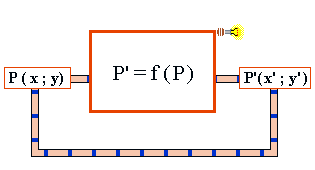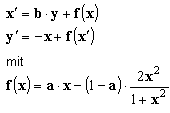### Simple Iterations

Here we use an Iteration Machine with a single function. The calculation starts with a point P (x; y) of the two-dimensional plane. The next point is calculated with a simple formula in two variables x and y. After that, we take the result P' (x'; y') and use it for the following calculation. This iteration is repeated until the plotted points build the attractor of the process.Here is the first example. Look for another one at page 2.

Mira Attractor Machine

Use the Mira machine by changing the parameter slides at the bottom of the image canvas. The new figure is immediately drawn. The table below shows sample values. The figures depend sensitively on the numerals behind the decimal point. The color is changed every 2000 dots, until the 10 predefined colors are used, then the colors are repeated.

The FormulaA

B

Scale

Name

0.29

1.0005

5

Fort

-0.48

0.93

10

Wing

-0.4

0.9999

7

Amoeba

-0.2

1.000

7

Carpet

Hints: Use -1 < A < 1 and B near 1